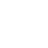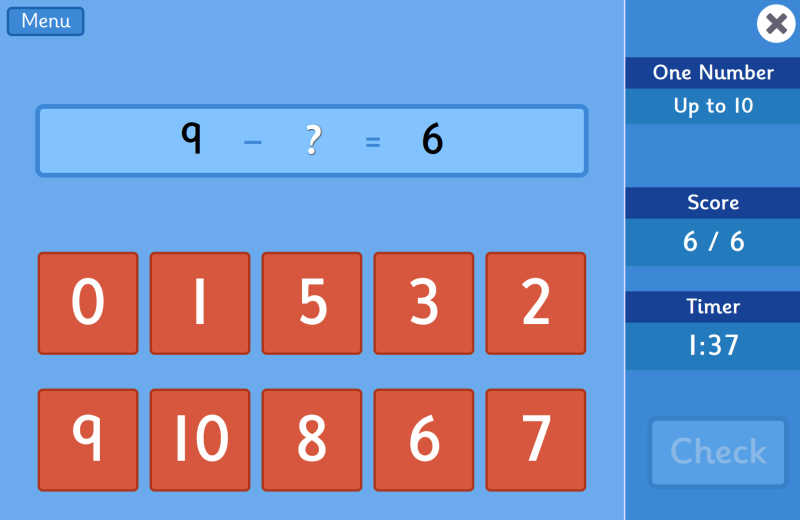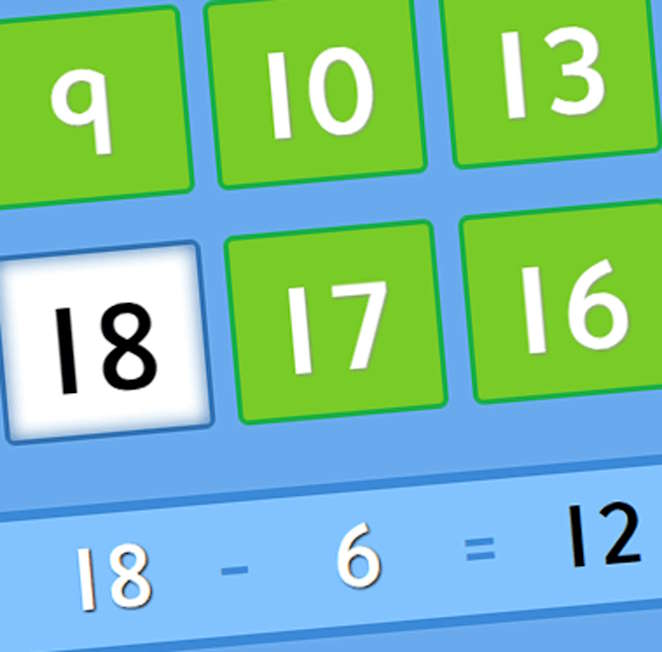# Subtraction Grids## QR CODE

Scan this on mobiles and tablets to quickly open this web page.Subtraction Grids is a mental maths game involving subtraction skills. It has two playing modes, where players need to select either one or two numbers from the grid to complete the subtraction calculation.

Each mode has six levels of difficulty and questions are randomised each time the games are played. The game is great for helping children improve their mental maths skills, especially those practising for SATs tests.

AGE
6-11 Years
SUBJECTS
Maths Number Subtraction# Subtraction Grids• One Number
• Two Numbers

## Pick one number to complete the subtraction calculation

• Up to 10
• Up to 20
• Up to 30
• Up to 100 (Tens)
• Up to 100
• Up to 2.0 (Decimals)

## Pick two numbers to complete the subtraction calculation

• Up to 10
• Up to 20
• Up to 30
• Up to 100 (Tens)
• Up to 100
• Up to 2.0 (Decimals)